• 旧车价格预测 某年没过旧车价格的调查资料如表1，其中 表示轿车的使用年数， 表示相应的平均价格，试分析用什么形式的曲线来拟合上述的数据，并预测使用4.5年后轿车的平均价格大致是多少?
• MATLAB程序分享MATLAB拟合求解圆心和半径源程序代码-MATLAB拟合求解圆心和半径 源程序代码.rar 程序代码见附件，拿资料请顺便顶个贴~~ 如果下载有问题，请加我 qq 1530497909，给你在线传
• 问题描述：如何利用matlab拟合?x=[503808.4 503793.6 503793.7 503793.7 503793.7 503793.8 503779.2 503779.2 503779.2 503779.2];>> y=[647086.5 647093.0 647107.9 647122.7 647137.1 647152.5 647160.6 ...
问题描述：如何利用matlab拟合?x=[503808.4 503793.6 503793.7 503793.7 503793.7 503793.8 503779.2 503779.2 503779.2 503779.2];>> y=[647086.5 647093.0 647107.9 647122.7 647137.1 647152.5 647160.6 647146.1 647145.3 647136.1];>> z=[150.786 150.99 151.136 151.655 151.444 151.053 150.241 150.59 150.36 150.363];>> z=a0+a1*x+a2*y+a3*x^2+a4*x*y+a5*y^2如何求a0,a1,a2,a3,a4,a51个回答分类：综合2014-10-04问题解答：我来补答clear;clc;x=[503808.4 503793.6 503793.7 503793.7 503793.7 503793.8 503779.2 503779.2 503779.2 503779.2];y=[647086.5 647093.0 647107.9 647122.7 647137.1 647152.5 647160.6 647146.1 647145.3 647136.1];z=[150.786 150.99 151.136 151.655 151.444 151.053 150.241 150.59 150.36 150.363];f1=@(x,y,p)(p(1)+p(2)*x+p(3)*y+p(4)*x.^2+p(5)*x.*y+p(6)*y.^2);f2=@(p)(sum((f1(x,y,p)-z).^2));fun=f2(sym('[p1 p2 p3 p4 p5 p6]'));[p1 p2 p3 p4 p5 p6]=solve(diff(fun,'p1'),diff(fun,'p2'),diff(fun,'p3'),diff(fun,'p4'),diff(fun,'p5'),diff(fun,'p6'));a=vpa([p1 p2 p3 p4 p5 p6],5)%这就是你要求的a0到a5p=double([p1 p2 p3 p4 p5 p6]);f=@(x,y)(f1(x,y,p));plot3(x,y,z,'o');hold on;ezsurf(f,[503770,503810,647080,647170])shading interp;alpha(.5);hold off;展开全文阅读
展开全文• matlab拟合曲线 matlab拟合曲线 matlab拟合曲线
• Matlab 拟合好坏常用指标 用过 Matlab拟合优化和统计等工具箱的网友会经常遇到下面几个名词 SSE(和方差误差平方和 ) The sum of squares due to error MSE(均方差方差 ) Mean squared error RMSE(均方根标准差 ) ...
• MATLAB拟合水土特征曲线，在matlab中很多拟合非线性曲线的方法，本文中介绍了用nlinfit和lsquurvefit两个函数拟合gardner曲线，效果很好。
• MATLAB拟合求圆心和半径，利用在excel表中的数据求出圆心坐标和半径大小，并标记显示。已运行成功，无错误。
• 为了满足智能仪表开发的需求，同时减轻开发人员的繁重工作，提出了利用Matlab拟合传感器特性曲线的办法，用该方法可迅速方便地建立传感器的数学模型。通过应用实例详细说了建立传感器数学模型的步骤。得出了数学模型...
• 这里记录一下自己使用matlab拟合的流程，也希望你太清楚matlab拟合用法的小伙伴能有所收获。 Curve Fitting工具1 打开工具2 用函数表达式拟合数据3 拟合设置4 自定义函数拟合5 无函数表达式的插值拟合6 导出拟合函数...
这两天也简单地用了matlab去拟合离散数据，感觉还是非常方便的。这里记录一下自己使用matlab拟合的流程，也希望你太清楚matlab拟合用法的小伙伴能有所收获。
Curve Fitting工具1 打开工具2 用函数表达式拟合数据3 拟合设置4 自定义函数拟合5 无函数表达式的插值拟合6 导出拟合函数（拟合关系）
1 打开工具
打开matlab，点击左上角的APP，找到Curve Fitting工作（当然打开它的方式有很多，不做展开）。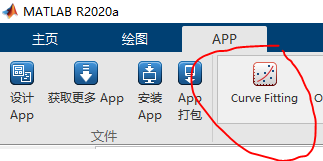打开以后的窗口如图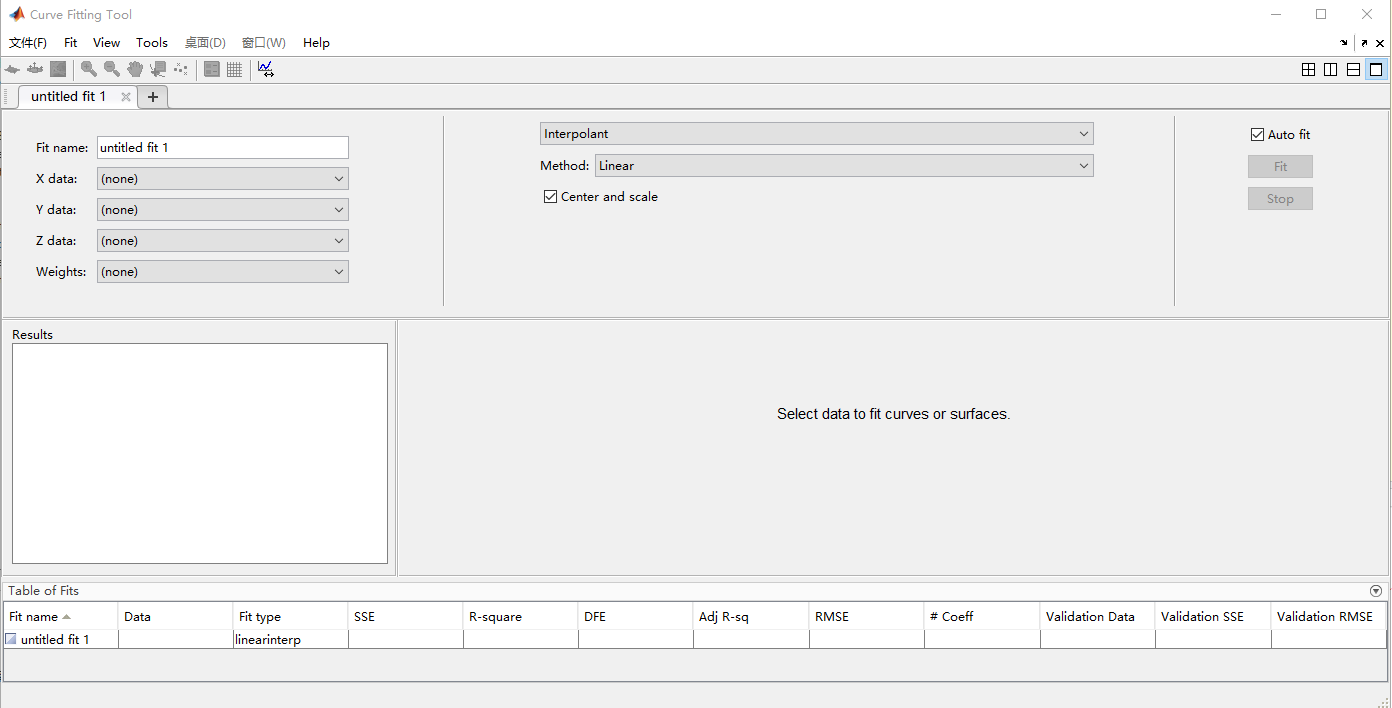这个窗口里有的选项也是超级多，但我会得不多，只是去摸索了一些够自己用的东西。
2 用函数表达式拟合数据
拟合数据前，肯定是要先有数据，因此咱们先做点数据。
x = 1:0.2:4;
y = x.^2 + rand(1,size(x,2));
plot(x,y,'+');

(x,y)就是我们创建的离散数据，数据的分布如下图：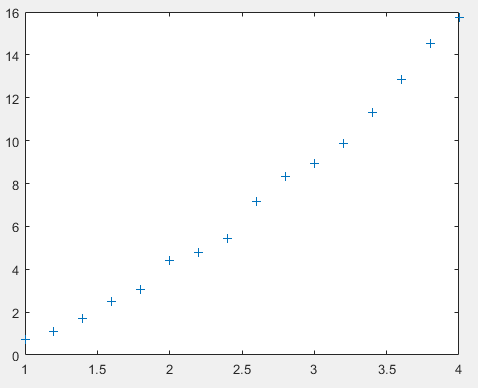因为加入了噪声，所以数据点的形状存在一些波动。那么现在用Curve Fitting工具来对这些离散数据做拟合。打开Curve Fitting工具，看到窗口的左边有【Fit name】、【X data】、【Y data】、【Z data】等等东西（我没有写的东西不是我忘写了，是我也不知道是干啥的）。
主要来看【X data】和【Y data】，这里是要输入要拟合的离散数据，那么【X data】就选刚刚创建的x序列，【Y data】就选刚刚船舰的y序列，画面上就会出现这些数据的分布了，如下图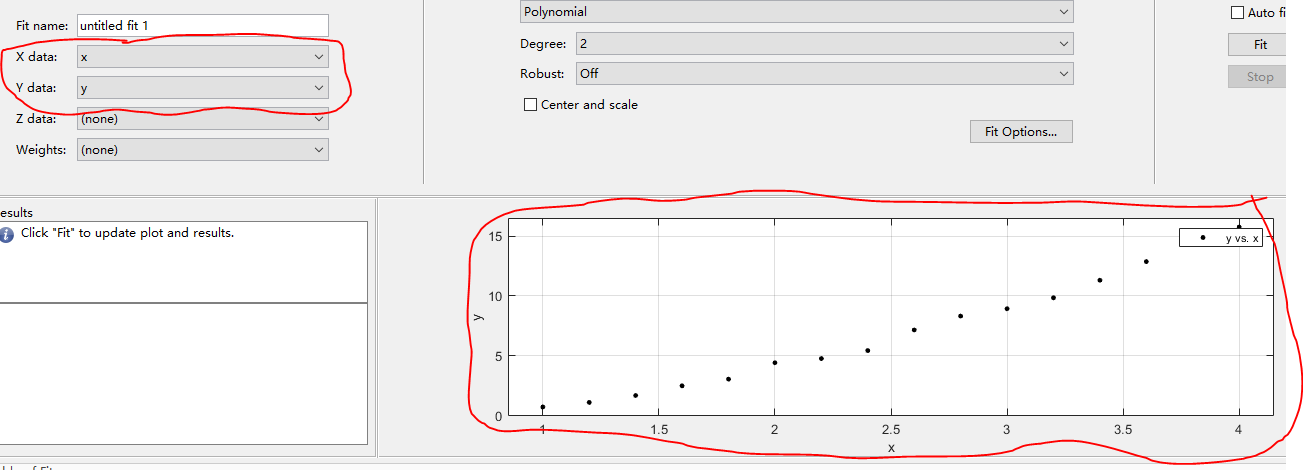然后看到窗口的中上部分，这里可以选择拟合的方式，关于拟合方式的详细介绍，大家可以看这篇文章，因为是人家整理的东西，我也不好直接复制过来。
各种拟合方式说明
这里，我们希望它能拟合成一个二次函数，所以选择【Polynomail(多项式拟合)】，【Degree（阶数）】选择为2，然后点击窗口右边的【fit】，就得到拟合的函数曲线和拟合的参数了。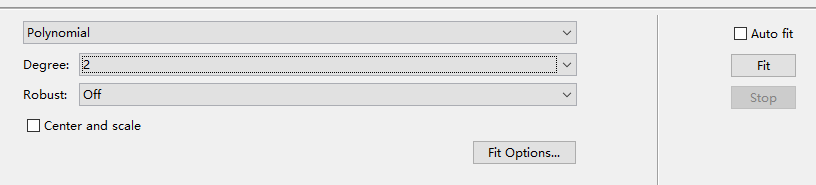拟合完以后，窗口上会显示的东西有，1）拟合的曲线；2）拟合后的参数；3）拟合误差的评估，如下图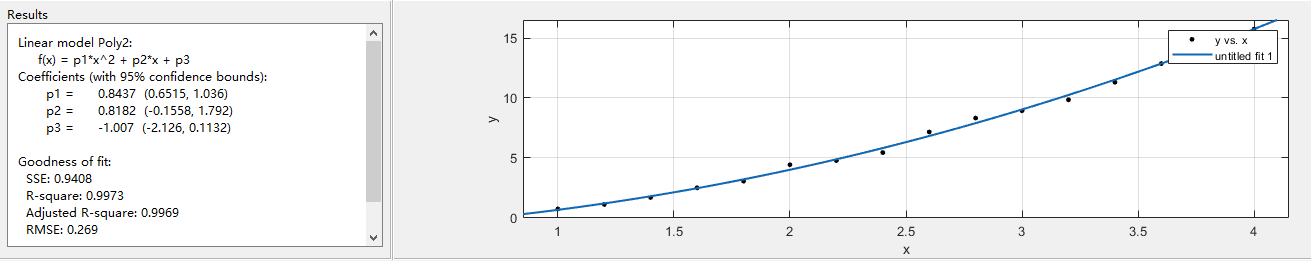可以看到（可能看不到，我图片都是复制的，不是导入的，应该巨糊……），给出的拟合结果是
f(x) = p1x^2 + p2x + p3，
p1 =0.8882 ，p2 =0.6097 ，p3 = -0.8021
这个拟合结果很垃圾，我们希望的结果应该是p1接近1，而p2和p3接近0。那么为什么会这样呢？首先第一个原因当然是因为加入了噪声的干扰，使得数据有偏差。其次，数据点太少了。
既然如此，那么就增多数据点，将x=1:0.2:4;改为x=1:0.01:4；数据一下增加了好多倍，而得到的拟合结果如下：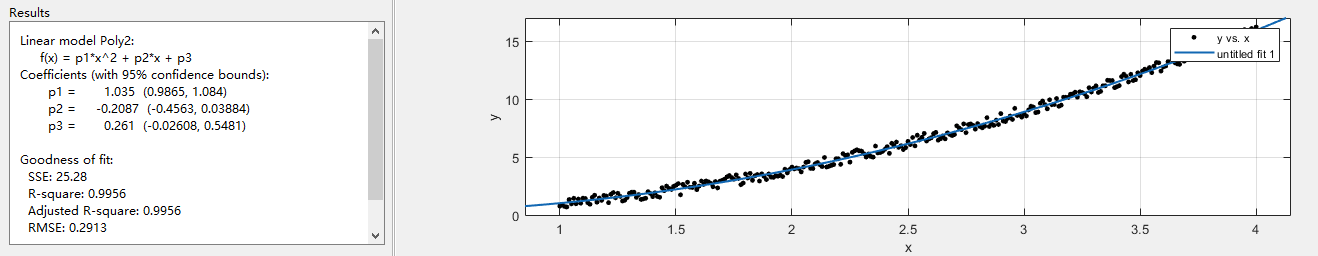拟合的结果是
f(x) = p1x^2 + p2x + p3，
p1 = 1.035，p2 = -0.2087，p3 =0.261
这个结果虽然还有不足，但比起第一次的结果又好很多了。那还有什么问题造成拟合效果不好呢？就是x的范围太小了，只有[1,4]，如果增加x的取值范围，那么拟合效果又会进一步提升。
上述只是一个很简单的例子，除了多项式拟合，这个工具还有很多其他的拟合方式，大家都可以尝试一下。
3 拟合设置
在窗口的中上位置，有一个【Fit Options】的按钮，点击它，就可以对拟合过程进行一些设置。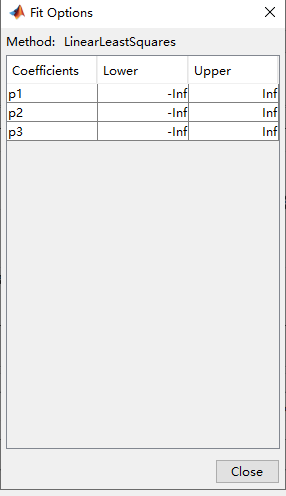我们上个例子是一个很简答的拟合过程，因此这里的拟合设置，可以控制的量也比较少，只有拟合参数的Lower(上限)和Upper(下限)设置。有些拟合方式还有又拟合参数的初值设置。有时候，设置一个好的处置和上下限，可以让拟合的结果收敛得更好。
这里只是和大家提一下有这个【Fit Options】设置，详细内容我知道也不多，就不展开了。
4 自定义函数拟合
在窗口得中上位置，选择拟合方式这里，可以选择【Custom Equation】，这个表示得是用户自定义一个函数进行拟合。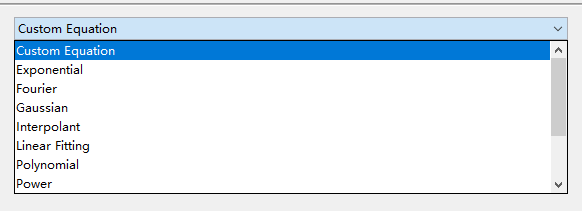我们先生成一些离散样本，这里，我们生成得样本y是一个Curve Fitting工具里面没有得一个拟合函数，离散样本的分布如下图。
x = 1:0.1:8;
y = sin(x)+log(x)+rand(1,71)-0.5;
plot(x,y,'+');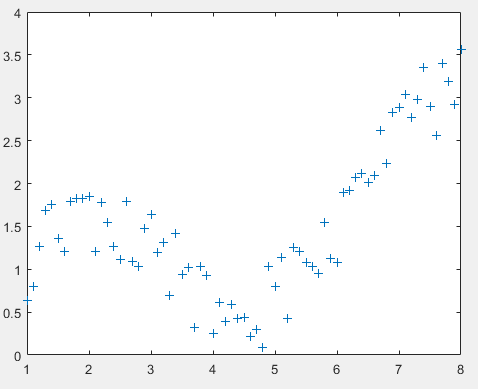我透，加了这个噪声以后，这啥也不是。不过好在我们知道我们要拟合的函数时什么样的。
选择【Custom Equation】的拟合方式，自定义一个拟合函数，如下图：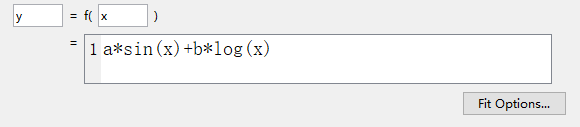我们定义的拟合函数是f(x)=asinx+blogx，这里的a与b就是拟合工具要给我们拟合出来的参数。
点击右侧的【Fit】，就可以得到拟合的曲线和拟合的参数了，如下图：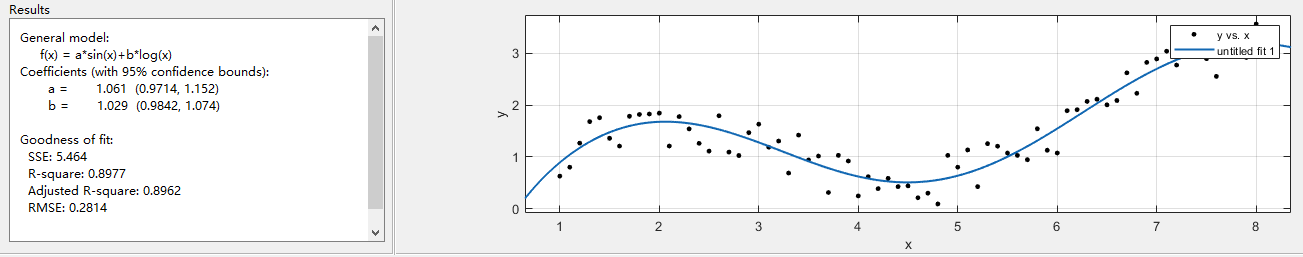拟合的结果为：
f(x) = asin(x)+blog(x)
a=1.061，b=1.029。
哦哟，拟合的效果竟然这么好？
5 无函数表达式的插值拟合
有些时候我们并不是需要一个准确的函数表达式，只是想由这些离散的数据，得到一个平滑的连续的曲线，那这时候可以用”插值“的方式来实现。
比如一个温度曲线，它是没有准确的函数表达式的，就是很多零散的观测点，而通过插值，我们可以得到哪些出在观测时间点中间时候的温度情况。
自行拟定一个温度的序列
x = 1:10;
y = [4,5,5,6,7,9,10,9.5,9,8.7];
plot(x,y,'+');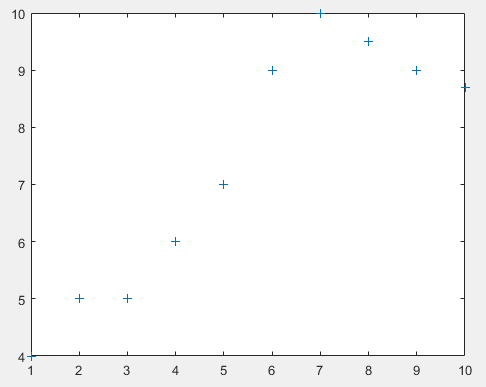这是一个温度变化的曲线，没有很准确的函数表达式，我们现在就是想通过插值拟合的方式，推测不是整点时间点的温度。
选择拟合方式为【Interpolant】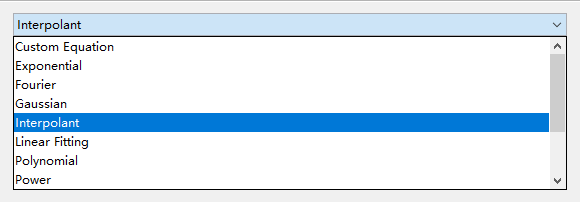在下栏的【Method】还可以选择插值的方式，这个我具体就不做展开了。这里我们选择【Shape-preserving(PCHIP】。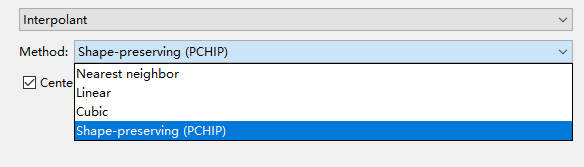点击右侧的【Fit】，就得到了拟合的曲线。这种拟合方式是没有函数表达式的，因此没有拟合的参数。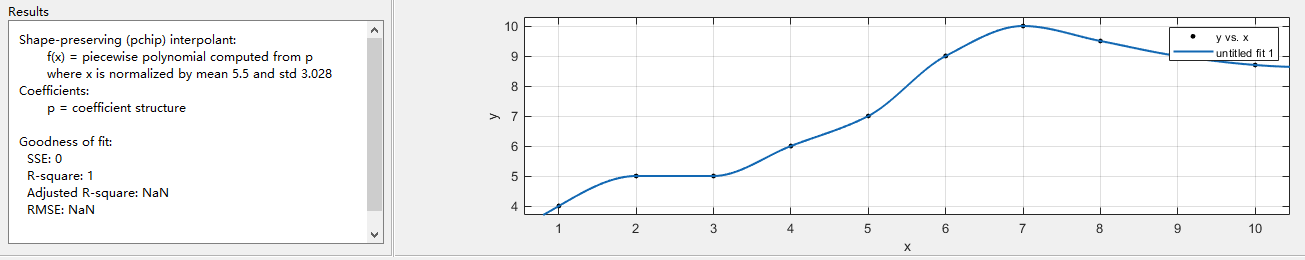6 导出拟合函数（拟合关系）
前面讲了3个例子，1）用一个二次多项式拟合；2）设置一个自定义函数去拟合；3）用插值的方式拟合了一条连续的曲线。
对于前两个，由于拟合的是有确定表达式的函数，在我们获得拟合参数以后，我们可以把这些参数套到函数里面去，就想怎么用就怎么用了。但对于第三种，它没有函数表达式，我们虽然拟合出来一个很好看的曲线，但用不上，怎么办呢？
其实，不管有没有函数表达式，拟合出来的拟合关系都是可以导出使用的。
当我们拟合出了一条曲线以后，点击Curve Fitting窗口右上角的【Fit】-【Sava to Workspace】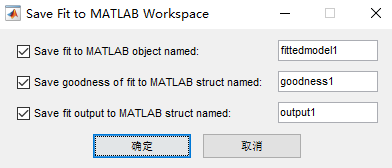上图的三个选项种，第一个表示导出拟合函数（拟合关系），剩下两个我没去研究过，就不做展开了。点击确定以后，拟合函数（拟合关系）就保存到工作区了。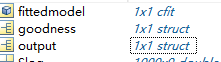主要cfit格式的数据，就是我们保存下来的拟合关系，双击它可以查看详情。我这里保存的是拟合温度变化的拟合关系，是插值拟合，所以没有函数关系，只写明了是PCHIP插值。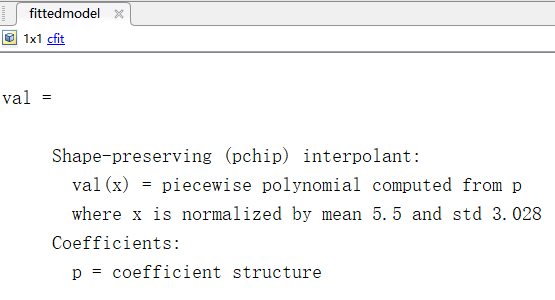那要怎么用这个拟合关系呢？它既然是一个关系，就需要自变量与因变量才能体现。如代码所演示：
% 设置自变量
xm=1:0.1:10;
% 将自变量套入刚刚保存的拟合关系里
ym=fittedmodel(xm);
plot(xm,ym,'+');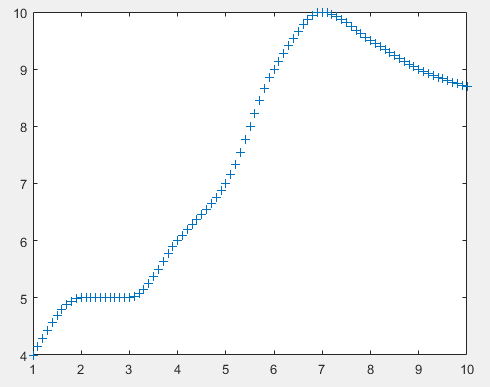欸，这就是我们刚刚保存下来的拟合关系了，就是自变量与因变量的关系了，这种关系虽然没有准确的表达式，但也是一种映射关系，所以也能称之为函数吧。
其实，这个工具就是用可视化操作的方式来进行拟合工作，而写代码，用函数，写参数，也是可以完成拟合工作，而且可以设置的选项还会更多一些。时间所限，今天就先记录一下这个Curve Fitting工具的使用，等后面有空，再补充一下fittype函数的使用，也是用来拟合数据的。


展开全文数据分析
• g"，点开"Curve Fitting Tool"，出现数据拟合工具界面，基本上所有的数据拟合和回归分析都可以在这里进行。也可以在命令窗口中直接输入”cftool”，打开工具箱。 2.输入两组向量 x，y。首先在 Matlab 的命令行输入两...
1.打开 CFTOOL 工具箱。g"，点开"Curve Fitting Tool"，出现数据拟合工具界面，基本上所有的数据拟合和回归分析都可以在这里进行。也可以在命令窗口中直接输入”cftool”，打开工具箱。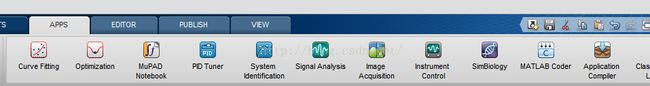2.输入两组向量 x，y。首先在 Matlab 的命令行输入两个向量，一个向量是你要的x坐标的各个数据，另外一个是你要的 y坐标的各个数据。输入以后假定叫 x向量与 y向量，可以在workspace 里面看见这两个向量，要确保这两个向量的元素数一致，如果不一致的话是不能在工具箱里面进行拟合的。例如在命令行里输入下列数据:x = [196,186, 137, 136, 122, 122, 71, 71, 70, 33];y=[0.012605,0.013115,0.016866,0.014741,0.022353,0.019278,0.041803,0.038026,0.038128,0.088196];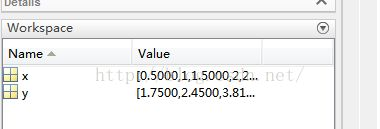3.数据的选取。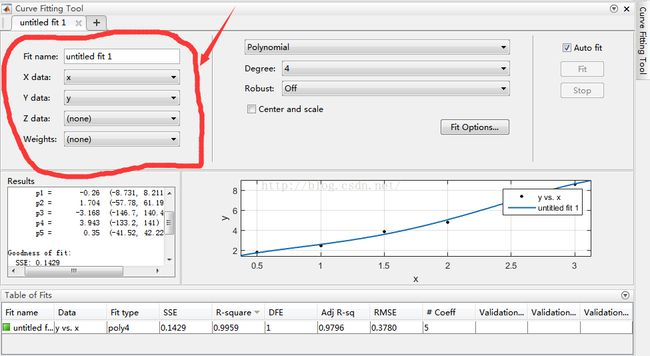4.曲线拟合(幂函数 power)。取拟合或回归类型，各个类型的拟合或回归相应的分别是：Custom Equations 用户自定义函数Expotential e指数函数Fourier 傅立叶函数，含有三角函数Gaussian 正态分布函数，高斯函数Interpolant 插值函数，含有线性函数，移动平均等类型的拟合Polynomial 多项式函数Power 幂函数Rational 有理函数Smooth Spline (光滑插值或者光滑拟合，不太清楚)Sum of sin functions 正弦函数类Weibull 威布尔函数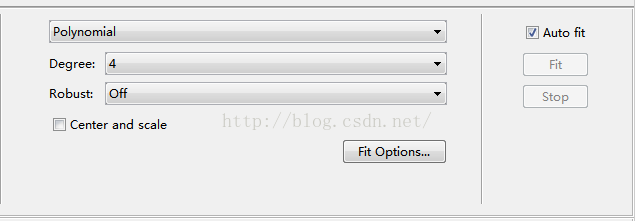5.拟合后的结果信息。在 Fitting对话框中的 Results文本框中显示有此次拟合的主要统计信息，主要有General model of sin1:....... (函数形式)Coefficients (with 95% conffidence range) (95%置信区间内的拟合常数)a1=... ( ... ...) (等号后面是平均值，括号里是范围)....Godness of fit: (统计结果)SSE: ... (方差)R-squared: ... (决定系数，不知道做什么的)Adjusted R-squared: ... (校正后的决定系数，如何校正的不得而知)RMSE: ... (标准差)for example:Linear model Poly4:f(x) = p1*x^4 + p2*x^3 + p3*x^2 + p4*x + p5Coefficients (with 95% confidence bounds):p1 =       -0.26  (-8.731, 8.211)p2 =       1.704  (-57.78, 61.19)p3 =      -3.168  (-146.7, 140.4)p4 =       3.943  (-133.2, 141)p5 =        0.35  (-41.52, 42.22)Goodness of fit:SSE: 0.1429R-square: 0.9959Adjusted R-square: 0.9796RMSE: 0.3787.图片导出。另外要说的是，如果想把这个拟合的图像导出的话，在 CurveFitting Tool窗口的 File菜单下选Print to Figure，此时弹出一个新的图像窗口，里面是你要导出的图像，在这个 figure 窗口的File菜单里再选 Export，选择好合适的格式，一般是 jpeg，选择好路径，点击 OK就可以了。出来的图像可以在Word等编辑环境中使用，就不多说了。要修改图像的性质，如数据点的大小、颜色等等的，只需要在对象上点右键，就差不多可以找到了。另外使用程序来进行曲线拟合：p=polyfit(xdata,ydata,n) n 为选取的方法a=polyval(p,xdata) 进行曲线拟合后计算所得到得值可以将拟合曲线与源曲线画出来：plot(xdata,ydata,'b*',xdata,a,'r-')legend('ydata','fit');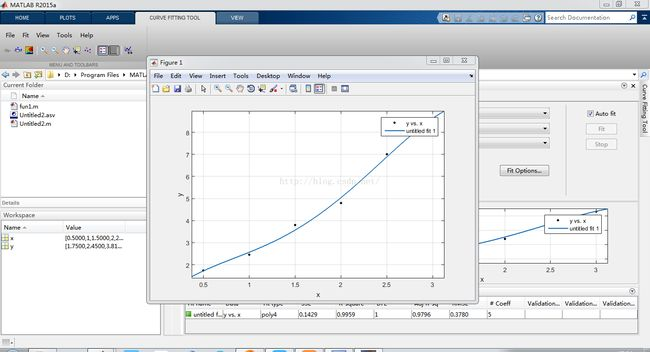展开全文• 使用matlab对数据进行简单的拟合，是只生成一个可以使所有点均匀分布在函数两侧，注意拟合阶数要适当，否则会产生高频震荡
• MATLAB拟合工具箱 cftool 用法及实例 1 2 3 4 分步阅读 我们利用MATLAB软件一个十分常用的功能就是进行曲线拟合，下面我就通过实例介绍一下 利用MATLAB拟合工具箱...


MATLAB拟合工具箱 cftool 用法及实例1234
分步阅读

我们利用MATLAB软件一个十分常用的功能就是进行曲线拟合，下面我就通过实例介绍一下 利用MATLAB拟合工具箱进行matlab曲线拟合的方法以及步骤。

工具/原料

MATLAB

方法/步骤

数据准备：

我们以一组多项式数据为例，进行示例，假如多项式是y=4x^3+3x^2+2产生的数据，x取0到3之间间隔为0.3的数。具体数据如下：调用工具箱：

关于如何调用工具箱我在其他经验中有详细的介绍，有兴趣的可以查看。

这里我们用命令cftool进行调用拟合工具箱，在MATLAB主窗口中输入 cftool 回车

可以看到如下拟合工具箱界面拟合操作步骤：

首先我们将要拟合的数据选入到工具箱中，如下图，在红圈处，点击向下三角，分别将要拟合的x y 选入，然后点击右侧的最上方的下三角，然后选择polynomial（ 多项式），下面的degree是阶数，也就是x的最高次数，选择不同的degree，在图的左下角是拟合的结果，包括拟合的系数以及方差相关系数等，右侧是数据点，以拟合曲线。结果分析：

我们拟合的时候，一般情况下不知道要拟合的多项式是几阶的，我们一般调节degree都是从1逐渐增大，只要精度符合要求，就可以了，并不是精度越高越高。

拟合结果说明：

Linear model Poly3:

f(x) = p1*x^3 + p2*x^2 + p3*x + p4

Coefficients (with 95% confidence bounds):

p1 =           4  (4, 4)

p2 =           3  (3, 3)

p3 =   4.593e-15  (-3.266e-14, 4.185e-14)

p4 =           2  (2, 2)

Goodness of fit:

SSE: 2.386e-28

R-square: 1

RMSE: 5.839e-15

从以上可以看到最终拟合的y关于x的函数为：

f(x)=4*x^3+3*x^2+4.593e-15*x+2

我们可以看到一次项的系数为4.593e-15，实际上就是4.593*10^（-15），这个数量级完全可以认为是0，所以拟合的结果我们认为是：

f(x)=4*x^3+3*x^2+2

这里的方差SSE数量级为10的负28次方，相关系数 R-square=1，说明拟合的结果很好。展开全文数学建模
• 一、打开matlab拟合工具箱 二、拟合工具箱的使用 三、自定义拟合函数——Custom Equation（建模用的较多） 四、多项式拟合（建模用的较多） 五、如何导出拟合的高清图像 六、调用拟合工具箱自动生成的代码 七...
• ## MATLAB拟合圆函数

千次阅读 2019-10-18 09:43:34
function [xc,yc,R,a] = ...%MATLAB拟合圆函数 n=length(x); xx=x.*x; yy=y.*y; xy=x.*y; A=[sum(x) sum(y) n;sum(xy) sum(yy)... sum(y);sum(xx) sum(xy) sum(x)]; B=[-sum(xx+yy) ; -sum(xx.*y+yy.*y) ; ...
• I am trying to fit a lognormal distribution using ... I've already done it using Matlab before but because of the need to extend the application beyond statistical analysis, I am in the process of ...
• 本技术手册描述了几种拟合给定数据集的曲线的方法，另外，本 手册还解释了加权曲线拟合、针对复数集的曲线拟合以及其他一些相关问题的拟合技巧。在介绍各种曲线拟合方法中，采用了典型例子的结合介绍。
• MATLAB拟合曲线的方法有很多，针对不同的函数类型应选用合适的拟合方法。 按照拟合方法来进行划分，一般可将待拟合的函数分为曲线/曲面拟合与多元函数拟合。 曲线/曲面拟合 曲线曲面拟合多是基于cftool拟合工具箱...可视化 数据分析
• matlab拟合圆 基于最小二乘法 详细推到过程.
• 土 壤 (Soils)201042(2)268-274 利用MATLAB拟合vanGenuchten方程参数的研究 杨改强 霍丽娟 杨国义 李一菲 钱天伟 (太原科技大学环境科学研究所太原 030024) 摘 要 选用 MATLAB的...8个函 数分别拟合
• 4.选择拟合方式 5.选择拟合时需要几阶？ 6.查看拟合效果 7.绘图显示 8.保存拟合数据 9.如何使用拟合曲线 1.打开方式 输入cftool 或者 2. 打开界面 3.输入数据 4.选择拟合方式 Custom Equations：...
• matlab拟合工具箱的使用,用于数学建模中方程拟合的工具包使用教程，你懂的！
• 通过调用matlab的gatool模块，用matlab拟合目标函数的参数。这是其中的M文件，使用时仅需稍作修改即可
• ## Matlab拟合幂律分布

千次阅读 2019-03-12 21:24:57
使用Matlab拟合幂律分布的两种方式： 一、编辑器输入 （1）新建matlab文件并输入对应的x,y变量（y为对应x值得概率） （2）点击运行后，右边工作区会显示对应x,y的变量 （3）点击菜单栏APP下的CurveFitting后...幂律分布...

# matlab拟合matlab 订阅